Courses

# Introduction - Turbulent Flow Mechanical Engineering Notes | EduRev

## Mechanical Engineering : Introduction - Turbulent Flow Mechanical Engineering Notes | EduRev

The document Introduction - Turbulent Flow Mechanical Engineering Notes | EduRev is a part of Mechanical Engineering category.
All you need of Mechanical Engineering at this link: Mechanical Engineering

Introduction

• The turbulent motion is an irregular motion.

• Turbulent fluid motion can be considered as an irregular condition of flow in which various quantities (such as velocity components and pressure) show a random variation with time and space in such a way that the statistical average of those quantities can be quantitatively expressed.

• It is postulated that the fluctuations inherently come from disturbances (such as roughness of a solid surface) and they may be either dampened out due to viscous damping or may grow by drawing energy from the free stream.

• At a Reynolds number less than the critical, the kinetic energy of flow is not enough to sustain the random fluctuations against the viscous damping and in such cases laminar flow continues to exist.

• At somewhat higher Reynolds number than the critical Reynolds number, the kinetic energy of flow supports the growth of fluctuations and transition to turbulence takes place.

Characteristics Of Turbulent Flow

• The most important characteristic of turbulent motion is the fact that velocity and pressure at a point fluctuate with time in a random manner.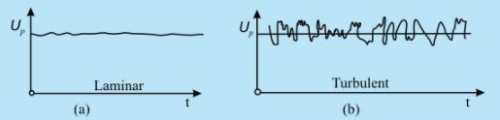Fig. 32.1 Variation of horizontal components of velocity for laminar and turbulent flows at a point P

• The mixing in turbulent flow is more due to these fluctuations. As a result we can see more uniform velocity distributions in turbulent pipe flows as compared to the laminar flows .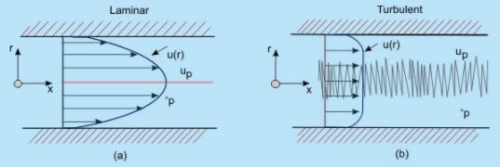Fig. 32.2 Comparison of velocity profiles in a pipe for (a) laminar and (b) turbulent flows

• Turbulence can be generated by -
1. frictional forces at the confining solid walls
2. the flow of layers of fluids with different velocities over one another

The turbulence generated in these two ways are considered to be different.

Turbulence generated and continuously affected by fixed walls is designated as wall turbulence , and turbulence generated by two adjacent layers of fluid in absence of walls is termed as free turbulence . One of the effects of viscosity on turbulence is to make the flow more homogeneous and less dependent on direction.

• Turbulence can be categorised as below -
• Homogeneous Turbulence: Turbulence has the same structure quantitatively in all parts of the flow field.
• Isotropic Turbulence: The statistical features have no directional preference and perfect disorder persists.
• Anisotropic Turbulence: The statistical features have directional preference and the mean velocity has a gradient.

• Homogeneous Turbulence : The term homogeneous turbulence implies that the velocity fluctuations in the system are random but the average turbulent characteristics are independent of the position in the fluid, i.e., invariant to axis translation.

Consider the root mean square velocity fluctuations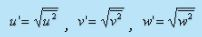In homogeneous turbulence, the rms values of u', v' and w' can all be different, but each value must be constant over the entire turbulent field. Note that even if the rms fluctuation of any component, say u' s are constant over the entire field the instantaneous values of u necessarily differ from point to point at any instant.

• Isotropic Turbulence: The velocity fluctuations are independent of the axis of reference, i.e. invariant to axis rotation and reflection. Isotropic turbulence is by its definition always homogeneous . In such a situation, the gradient of the mean velocity does not exist, the mean velocity is either zero or constant throughout.

In isotropic turbulence fluctuations are independent of the direction of reference and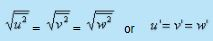It is re-emphasised that even if the rms fluctuations at any point are same, their instantaneous values necessarily differ from each other at any instant.

• Turbulent flow is diffusive and dissipative . In general, turbulence brings about better mixing of a fluid and produces an additional diffusive effect. Such a diffusion is termed as "Eddy-diffusion ".( Note that this is different from molecular diffusion)
At a large Reynolds number there exists a continuous transport of energy from the free stream to the large eddies. Then, from the large eddies smaller eddies are continuously formed. Near the wall smallest eddies destroy themselves in dissipating energy, i.e., converting kinetic energy of the eddies into intermolecular energy.

Laminar-Turbulent Transition

• For a turbulent flow over a flat plate,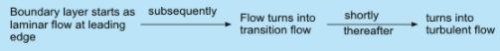The turbulent boundary layer continues to grow in thickness, with a small region below it called a viscous sublayer. In this sub layer, the flow is well behaved,just as the laminar boundary layer (Fig. 32.3)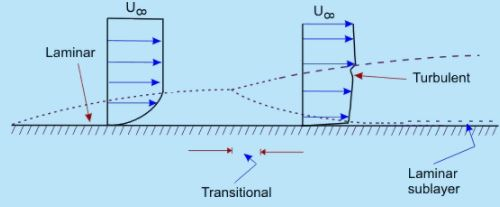• Fig. 32.3 Laminar - turbulent transition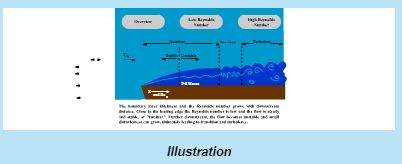• Observe that at a certain axial location, the laminar boundary layer tends to become unstable. Physically this means that the disturbances in the flow grow in amplitude at this location.

Free stream turbulence, wall roughness and acoustic signals may be among the sources of such disturbances. Transition to turbulent flow is thus initiated with the instability in laminar flow

• The possibility of instability in boundary layer was felt by Prandtl as early as 1912.The theoretical analysis of Tollmien and Schlichting showed that unstable waves could exist if the Reynolds number was 575.

The Reynolds number was defined as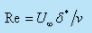where U is the free stream velocity , δ* is the displacement thickness and ν is the kinematic viscosity .

• Taylor developed an alternate theory, which assumed that the transition is caused by a momentary separation at the boundary layer associated with the free stream turbulence.
In a pipe flow the initiation of turbulence is usually observed at Reynolds numbers (U D/v )in the range of 2000 to 2700.

The development starts with a laminar profile, undergoes a transition, changes over to turbulent profile and then stays turbulent thereafter   (Fig. 32.4). The length of development is of the order of 25 to 40 diameters of the pipe.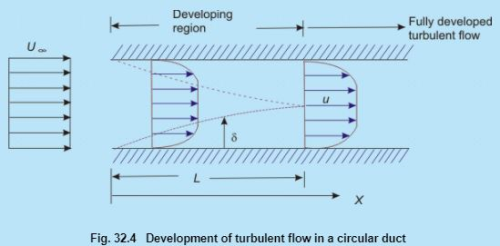Correlation Functions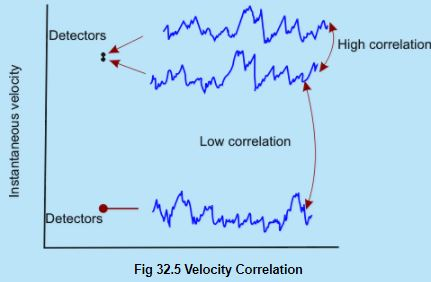• A statistical correlation can be applied to fluctuating velocity terms in turbulence. Turbulent motion is by definition eddying motion. Not withstanding the circulation strength of the individual eddies, a high degree of correlation exists between the velocities at two points in space, if the distance between the points is smaller than the diameter of the eddy. Conversely, if the points are so far apart that the space, in between, corresponds to many eddy diameters (Figure 32.5), little correlation can be expected.

• Consider a statistical property of a random variable (velocity) at two points separated by a distance r. An Eulerian correlation tensor (nine terms) at the two points can be defined by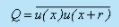In other words, the dependence between the two velocities at two points is measured by the correlations, i.e. the time averages of the products of the quantities measured at two points. The correlation of the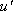components of the turbulent velocity of these two points is defined as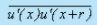It is conventional to work with the non-dimensional form of the correlation, such as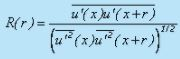A value of R(r) of unity signifies a perfect correlation of the two quantities involved and their motion is in phase.Negative value of the correlation function implies that the time averages of the velocities in the two correlated points have different signs. Figure 32.6 shows typical variations of the correlation R with increasing separation r .

The positive correlation indicates that the fluid can be modelled as travelling in lumps. Since swirling motion is an essential feature of turbulent motion, these lumps are viewed as eddies of various sizes. The correlation R(r) is a measure of the strength of the eddies of size larger than r. Essentially the velocities at two points are correlated if they are located on the same eddy

• To describe the evolution of a fluctuating function u'(t), we need to know the manner in which the value of u' at different times are related. For this purpose the correlation function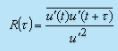between the values of u' at different times is chosen and is called autocorrelation function.

• The correlation studies reveal that the turbulent motion is composed of eddies which are convected by the mean motion . The eddies have a wide range variation in their size. The size of the large eddies is comparable with the dimensions of the neighbouring objects or the dimensions of the flow passage.

The size of the smallest eddies can be of the order of 1 mm or less. However, the smallest eddies are much larger than the molecular mean free paths and the turbulent motion does obey the principles of continuum mechanics.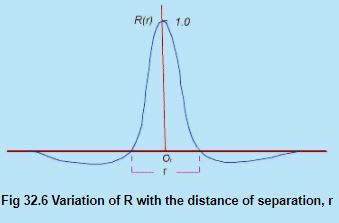Reynolds decomposition of turbulent flow :

• The Experiment: In 1883, O. Reynolds conducted experiments with pipe flow by feeding into the stream a thin thread of liquid dye. For low Reynolds numbers, the dye traced a straight line and did not disperse. With increasing velocity, the dye thread got mixed in all directions and the flowing fluid appeared to be uniformly colored in the downstream flow.

The Inference: It was conjectured that on the main motion in the direction of the pipe axis, there existed a superimposed motion all along the main motion at right angles to it. The superimposed motion causes exchange of momentum in transverse direction and the velocity distribution over the cross-section is more uniform than in laminar flow. This description of turbulent flow which consists of superimposed streaming and fluctuating (eddying) motion is well known as Reynolds decomposition of turbulent flow.

• Here, we shall discuss different descriptions of mean motion. Generally, for Eulerian velocity u , the following two methods of averaging could be obtained.

(i) Time average for a stationary turbulence: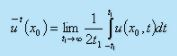(ii) Space average for a homogeneous turbulence: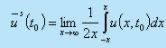For a stationary and homogeneous turbulence, it is assumed that the two averages lead to the same result: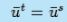and the assumption is known as the ergodic hypothesis.

• In our analysis, average of any quantity will be evaluated as a time average . Take a finite time interval t1. This interval must be larger than the time scale of turbulence. Needless to say that it must be small compared with the period t2 of any slow variation (such as periodicity of the mean flow) in the flow field that we do not consider to be chaotic or turbulent .

Thus, for a parallel flow, it can be written that the axial velocity component is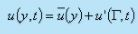As such, the time mean component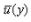determines whether the turbulent motion is steady or not. The symbol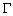signifies any of the space variables.

• While the motion described by Fig.32.6(a) is for a turbulent flow with steady mean velocity the Fig.32.6(b) shows an example of turbulent flow with unsteady mean velocity. The time period of the high frequency fluctuating component is t1 whereas the time period for the unsteady mean motion is t2 and for obvious reason t2>>t1. Even if the bulk motion is parallel, the fluctuation ' being random varies in all directions.
• The continuity equation, gives us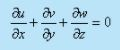Invoking Eq.(32.1) in the above expression, we ge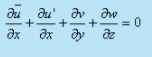(32.2)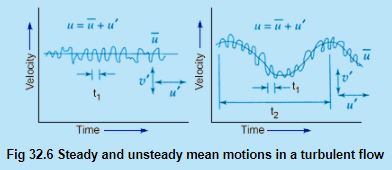Since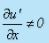, Eq.(32.2) depicts that and components of velocity exist even for the parallel flow if the flow is turbulent. We have-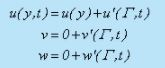(32.3)

Contd. from Previous slide

• However, the fluctuating components do not bring about the bulk displacement of a fluid element. The instantaneous displacement is u ' dt , and that is not responsible for the bulk motion. We can conclude from the above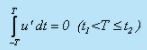Due to the interaction of fluctuating components, macroscopic momentum transport takes place. Therefore, interaction effect between two fluctuating components over a long period is non-zero and this can be expressed as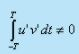Taking time average of these two integrals and write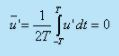(32.4a)

and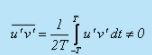(32.4b)

• Now, we can make a general statement with any two fluctuating parameters, say, with ' and g' as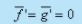(32.5a)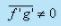(32.5b)

The time averages of the spatial gradients of the fluctuating components also follow the same laws, and they can be written as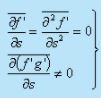(32.6

• The intensity of turbulence or degree of turbulence in a flow is described by the relative magnitude of the root mean square value of the fluctuating components with respect to the time averaged main velocity. The mathematical expression is given by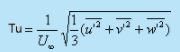(32.7a)

The degree of turbulence in a wind tunnel can be brought down by introducing screens of fine mesh at the bell mouth entry. In general, at a certain distance from the screens, the turbulence in a wind tunnel becomes isotropic, i.e. the mean oscillation in the three components are equal,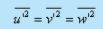In this case, it is sufficient to consider the oscillation u' in the direction of flow and to put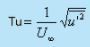(32.7b)

This simpler definition of turbulence intensity is often used in practice even in cases when turbulence is not isotropic.

Following Reynolds decomposition, it is suggested to separate the motion into a mean motion and a fluctuating or eddying motion. Denoting the time average of the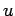component of velocity by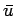and fluctuating component as  u' we can write down the following,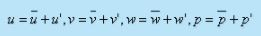By definition, the time averages of all quantities describing fluctuations are equal to zero.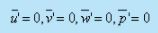(32.8)

The fluctuations u', v' , and w' influence the mean motion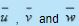in such a way that the mean motion exhibits an apparent increase in the resistance to deformation. In other words, the effect of fluctuations is an apparent increase in viscosity or macroscopic momentum diffusivity .

• Rules of mean time - averages

If and g are two dependent variables and if s denotes anyone of the independent variables x, y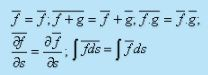Offer running on EduRev: Apply code STAYHOME200 to get INR 200 off on our premium plan EduRev Infinity!

,

,

,

,

,

,

,

,

,

,

,

,

,

,

,

,

,

,

,

,

,

;